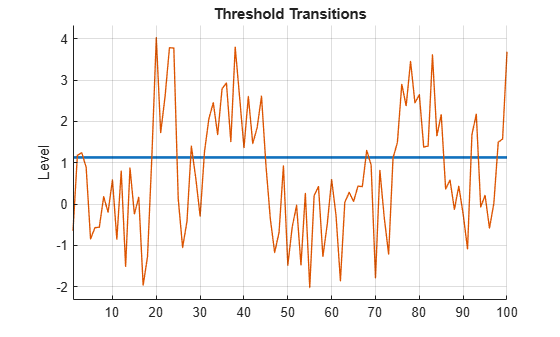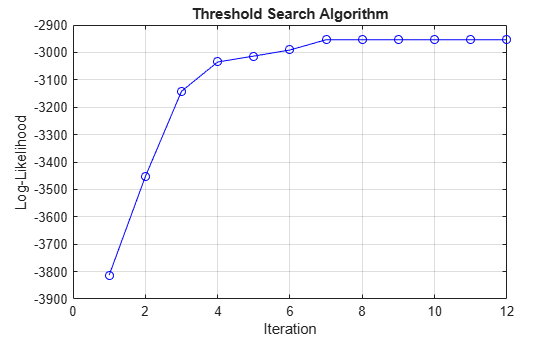# summarize

Summarize threshold-switching dynamic regression model estimation results

## Syntax

``summarize(Mdl)``
``summarize(Mdl,state)``
``results = summarize(___)``

## Description

example

````summarize(Mdl)` displays a summary of the threshold-switching dynamic regression model `Mdl`. If `Mdl` is an estimated model returned by `estimate`, then `summarize` displays estimation results to the MATLAB® Command Window. The display includes: A model descriptionEstimated threshold transitionsFit statistics, which include the effective sample size, number of estimated submodel parameters and constraints, loglikelihood, and information criteria (AIC and BIC)A table of submodel estimates and inferences, which includes coefficient estimates with standard errors, t-statistics, and p-values If `Mdl` is an unestimated threshold-switching model returned by `tsVAR`, `summarize` prints the standard object display (the same display that `tsVAR` prints during model creation). ```

example

````summarize(Mdl,state)` displays only summary information for the submodel with name `state`.```

example

````results = summarize(___)` returns one of the following variables using any of the input argument combinations in the previous syntaxes. If `Mdl` is an estimated threshold-switching model, `results` is a table containing the submodel estimates and inferences.If `Mdl` is an unestimated model, `results` is a `tsVAR` object that is equal to `Mdl`. `summarize` does not print to the Command Window```

## Examples

collapse all

Assess estimation accuracy using simulated data from a known data-generating process (DGP). This example uses arbitrary parameter values.

Create Model for DGP

Create a discrete threshold transition at mid-level `1`.

`ttDGP = threshold(1)`
```ttDGP = threshold with properties: Type: 'discrete' Levels: 1 Rates: [] StateNames: ["1" "2"] NumStates: 2 ```

`ttDGP` is a `threshold` object representing the state-switching mechanism of the DGP.

Create the following fully specified self-exciting TAR (SETAR) model for the DGP.

• State 1: ${\mathit{y}}_{\mathit{t}}={\epsilon }_{\mathit{t}}$.

• State 2: ${\mathit{y}}_{\mathit{t}}=2+{\epsilon }_{\mathit{t}}$.

• ${\epsilon }_{\mathit{t}}\sim Ν\left(0,1\right)$.

Specify the submodels by using `arima`.

```mdl1DGP = arima(Constant=0); mdl2DGP = arima(Constant=2); mdlDGP = [mdl1DGP mdl2DGP];```

Because the innovations distribution is invariant across states, the `tsVAR` software ignores the value of the submodel innovations variance (`Variance` property).

Create a threshold-switching model for the DGP. Specify the model-wide innovations variance.

`MdlDGP = tsVAR(ttDGP,mdlDGP,Covariance=1);`

`MdlDGP` is a `tsVAR` object representing the DGP.

Simulate Response Paths from DGP

Generate a random response path of length 100 from the DGP. By default, `simulate` assumes a SETAR model with delay $\mathit{d}=1$. In other words, the threshold variable is ${\mathit{y}}_{\mathit{t}-1}$.

```rng(1) % For reproducibiliy y = simulate(MdlDGP,100);```

`y` is a 100-by-1 vector of representing the simulated response path.

Create Model for Estimation

Create a partially specified threshold-switching model that has the same structure as the data-generating process, but specify the transition mid-level, submodel coefficients, and model-wide constant as unknown for estimation.

```tt = threshold(NaN); mdl1 = arima('Constant',NaN); mdl2 = arima('Constant',NaN); Mdl = tsVAR(tt,[mdl1,mdl2],'Covariance',NaN);```

`Mdl` is a partially specified `tsVAR` object representing a template for estimation. `NaN`-valued elements of the `Switch` and `Submodels` properties indicate estimable parameters.

`Mdl` is agnostic of the threshold variable; `tsVAR` object functions enable you to specify threshold variable characteristics or data.

Create Threshold Transitions Containing Initial Values

The estimation procedure requires initial values for all estimable threshold transition parameters.

Fully specify a threshold transition that has the same structure as `tt`, but set the mid-level to `0`.

`tt0 = threshold(0);`

`tt0` is a fully specified `threshold` object.

Estimate Model

Fit the model to the simulated path. By default, the model is self-exciting and the delay of the threshold variable is $\mathit{d}=1$.

`EstMdl = estimate(Mdl,tt0,y)`
```EstMdl = tsVAR with properties: Switch: [1x1 threshold] Submodels: [2x1 varm] NumStates: 2 NumSeries: 1 StateNames: ["1" "2"] SeriesNames: "1" Covariance: 1.0225 ```

`EstMdl` is a fully specified `tsVAR` object representing the estimated SETAR model.

Display an estimation summary of the submodels.

`summarize(EstMdl)`
```Description 1-Dimensional tsVAR Model with 2 Submodels Switch Transition Type: discrete Estimated Levels: 1.128 Fit Effective Sample Size: 99 Number of Estimated Parameters: 2 Number of Constrained Parameters: 0 LogLikelihood: -141.574 AIC: 287.149 BIC: 292.339 Submodels Estimate StandardError TStatistic PValue ________ _____________ __________ __________ State 1 Constant(1) -0.12774 0.13241 -0.96474 0.33467 State 2 Constant(1) 2.1774 0.16829 12.939 2.7264e-38 ```

The estimates are close to their true values.

Plot the estimated switching mechanism with the threshold data, which is the response data.

```figure ttplot(EstMdl.Switch,'Data',y)```Create the following fully specified SETAR model for the DGP.

• State 1: $\left[\begin{array}{c}{y}_{1,t}\\ {y}_{2,t}\end{array}\right]=\left[\begin{array}{c}-1\\ -4\end{array}\right]+\left[\begin{array}{cc}-0.5& 0.1\\ 0.2& -0.75\end{array}\right]\left[\begin{array}{c}{y}_{1,t-1}\\ {y}_{2,t-1}\end{array}\right]+\left[\begin{array}{c}{\epsilon }_{1,t}\\ {\epsilon }_{2,t}\end{array}\right]$.

• State 2: $\left[\begin{array}{c}{y}_{1,t}\\ {y}_{2,t}\end{array}\right]=\left[\begin{array}{c}1\\ 4\end{array}\right]+\left[\begin{array}{c}{\epsilon }_{1,t}\\ {\epsilon }_{2,t}\end{array}\right]$.

• State 3:$\left[\begin{array}{c}{y}_{1,t}\\ {y}_{2,t}\end{array}\right]=\left[\begin{array}{c}1\\ 4\end{array}\right]+\left[\begin{array}{cc}0.5& 0.1\\ 0.2& 0.75\end{array}\right]\left[\begin{array}{c}{y}_{1,t-1}\\ {y}_{2,t-1}\end{array}\right]+\left[\begin{array}{c}{\epsilon }_{1,t}\\ {\epsilon }_{2,t}\end{array}\right]$.

• $\left[\begin{array}{c}{\epsilon }_{1,t}\\ {\epsilon }_{2,t}\end{array}\right]\sim {N}_{2}\left(\left[\begin{array}{c}0\\ 0\end{array}\right],\left[\begin{array}{cc}2& -1\\ -1& 1\end{array}\right]\right)$.

• The system is in state 1 when ${\mathit{y}}_{2,\mathit{t}-4}<-3$, the system is in state 2 when $-3\le {\mathit{y}}_{2,\mathit{t}-4}<3$, and the system is in state 3 otherwise.

```t = [-3 3]; ttDGP = threshold(t); constant1 = [-1; -4]; constant2 = [1; 4]; constant3 = [1; 4]; AR1 = [-0.5 0.1; 0.2 -0.75]; AR3 = [0.5 0.1; 0.2 0.75]; Sigma = [2 -1; -1 1]; mdl1DGP = varm(Constant=constant1,AR={AR1}); mdl2DGP = varm(Constant=constant2); mdl3DGP = varm(Constant=constant3,AR={AR3}); mdlDGP = [mdl1DGP; mdl2DGP; mdl3DGP]; MdlDGP = tsVAR(ttDGP,mdlDGP,Covariance=Sigma);```

DIsplay a summary of the unestimated DGP.

`summarize(MdlDGP)`
```Mdl = tsVAR with properties: Switch: [1x1 threshold] Submodels: [3x1 varm] NumStates: 3 NumSeries: 2 StateNames: ["1" "2" "3"] SeriesNames: ["1" "2"] Covariance: [2x2 double] ```

`summarize` prints an object display.

Generate a random response path of length 500 from the DGP. Specify that second response variable with a delay of 4 as the threshold variable.

```rng(10) % For reproducibiliy y = simulate(MdlDGP,500,Index=2,Delay=4);```

Create a partially specified threshold-switching model that has the same structure as the DGP, but specify the transition mid-level, submodel coefficients, and model-wide covariance as unknown for estimation.

```tt = threshold([NaN; NaN]); mdlar = varm(2,1); mdlc = varm(2,0); Mdl = tsVAR(tt,[mdlar; mdlc; mdlar],Covariance=nan(2));```

Fully specify a threshold transition that has the same structure as `tt`, but set the mid-levels to -1 and 1.

```t0 = [-1 1]; tt0 = threshold(t0);```

Fit the threshold-switching model to the simulated series. Specify the threshold variable ${\mathit{y}}_{2,\mathit{t}-4}$. Plot the loglikelihood after each iteration of the threshold search algorithm.

`EstMdl = estimate(Mdl,tt0,y,IterationPlot=true,Index=2,Delay=4);`The plot displays the evolution of the loglikelihood as the estimation procedure searches for optimal levels. The procedure terminates when one of the stopping criteria is satisfied.

Display an estimation summary for state 3 only.

`summarize(EstMdl,3)`
```Description 2-Dimensional VAR Submodel, State 3 Submodel Estimate StandardError TStatistic PValue ________ _____________ __________ __________ State 3 Constant(1) 1.0621 0.095701 11.098 1.2802e-28 State 3 Constant(2) 3.8707 0.068772 56.284 0 State 3 AR{1}(1,1) 0.47396 0.058016 8.1694 3.0997e-16 State 3 AR{1}(2,1) 0.23013 0.041691 5.5199 3.3927e-08 State 3 AR{1}(1,2) 0.10561 0.018233 5.7924 6.9371e-09 State 3 AR{1}(2,2) 0.7568 0.013102 57.761 0 ```

Create a discrete threshold transition at mid-level 1.

`ttDGP = threshold(1)`
```ttDGP = threshold with properties: Type: 'discrete' Levels: 1 Rates: [] StateNames: ["1" "2"] NumStates: 2 ```

Create the following fully specified self-exciting TAR (SETAR) model for the DGP.

• State 1: ${\mathit{y}}_{\mathit{t}}={\epsilon }_{\mathit{t}}$.

• State 2: ${\mathit{y}}_{\mathit{t}}=2+{\epsilon }_{\mathit{t}}.$

• ${\epsilon }_{\mathit{t}}\sim Ν\left(0,1\right)$.

Specify the submodels by using `arima`.

```mdl1DGP = arima(Constant=0); mdl2DGP = arima(Constant=2); mdlDGP = [mdl1DGP mdl2DGP];```

Create a threshold-switching model for the DGP. Specify the model-wide innovations variance.

`MdlDGP = tsVAR(ttDGP,mdlDGP,Covariance=1);`

Generate a random response path of length 100 from the DGP. By default, `simulate` assumes a SETAR model with delay $\mathit{d}$ = 1. In other words, the threshold variable is ${\mathit{y}}_{\mathit{t}-1}$.

```rng(1) % For reproducibiliy y = simulate(MdlDGP,100);```

Create a partially specified threshold-switching model that has the same structure as the data-generating process, but specify the transition mid-level, submodel coefficients, and model-wide constant as unknown for estimation.

```tt = threshold(NaN); mdl1 = arima('Constant',NaN); mdl2 = arima('Constant',NaN); Mdl = tsVAR(tt,[mdl1,mdl2],'Covariance',NaN);```

Fully specify a threshold transition that has the same structure as `tt`, but set the mid-level to 0.

`tt0 = threshold(0);`

Fit the model to the simulated path. By default, the model is self-exciting and the delay of the threshold variable is $\mathit{d}=1$.

`EstMdl = estimate(Mdl,tt0,y);`

Return an estimation summary table.

`results = summarize(EstMdl)`
```results=2×4 table Estimate StandardError TStatistic PValue ________ _____________ __________ __________ State 1 Constant(1) -0.12774 0.13241 -0.96474 0.33467 State 2 Constant(1) 2.1774 0.16829 12.939 2.7264e-38 ```

`results` is a table containing estimates and inferences for all submodel coefficients.

Identify significant coefficient estimates.

`results.Properties.RowNames(results.PValue < 0.05)`
```ans = 1x1 cell array {'State 2 Constant(1)'} ```

## Input Arguments

collapse all

Threshold-switching dynamic regression model, specified as a `tsVAR` object returned by `estimate` or `tsVAR`.

State to summarize, specified as an integer in `1:Mdl.NumStates` or a state name in `Mdl.StateNames`.

The default summarizes all states.

Example: `summarize(Mdl,3)` summarizes the third state in `Mdl`.

Example: `summarize(Mdl,"Recession")` summarizes the state labeled `"Recession"` in `Mdl`.

Data Types: `double` | `char` | `string`

## Output Arguments

collapse all

Model summary, returned as a table or `tsVAR` object.

• If `Mdl` is an estimated threshold-switching model returned by `estimate`, `results` is a table of summary information for the submodel parameter estimates. Each row corresponds to a submodel coefficient. Columns correspond to the estimate (`Estimate`), standard error (`StandardError`), t-statistic (`TStatistic`), and the p-value (`PValue`).

When the summary includes all states (the default), `results.Properties` stores the following fit statistics:

FieldDescription
`Description`Model summary description (character vector)
`EffectiveSampleSize`Effective sample size (numeric scalar)
`NumEstimatedParameters`Number of estimated parameters (numeric scalar)
`NumConstraints`Number of equality constraints (numeric scalar)
`LogLikelihood`Optimized loglikelihood value (numeric scalar)
`AIC`Akaike information criterion (numeric scalar)
`BIC`Bayesian information criterion (numeric scalar)

• If `Mdl` is an unestimated model, `results` is a `tsVAR` object that is equal to `Mdl`.

Note

When `results` is a table, it contains only submodel parameter estimates:

• `Mdl.Switch` contains estimates of threshold transitions.

• Threshold-switching models can have one or more residual covariance matrices. When `Mdl` has a model-wide covariance, `Mdl.Covariance` contains the estimated residual covariance. Otherwise, `Mdl.Submodels(j).Covariance` contains the estimated residual covariance of state `j`. For details, see `tsVAR`.

## Algorithms

`estimate` searches over levels and rates for estimated threshold transitions while solving a conditional least-squares problem for submodel parameters, as described in . The standard errors, loglikelihood, and information criteria are conditional on optimal parameter values in the estimated threshold transitions `Mdl.Switch`. In particular, standard errors do not account for variation in estimated levels and rates.

 Teräsvirta, Tima. "Modelling Economic Relationships with Smooth Transition Regressions." In A. Ullahand and D.E.A. Giles (eds.), Handbook of Applied Economic Statistics, 507–552. New York: Marcel Dekker, 1998.

 van Dijk, Dick. Smooth Transition Models: Extensions and Outlier Robust Inference. Rotterdam, Netherlands: Tinbergen Institute Research Series, 1999.